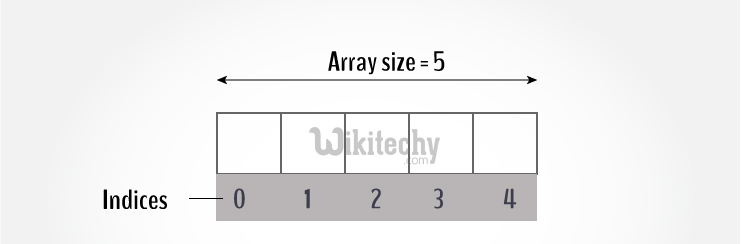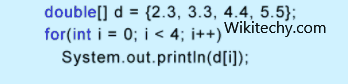# golang tutorial - Golang Array | Go arrays - golang - go programming language - google go - go language - go program - google language

## Golang Array

• In Go, an array is a numbered sequence of elements of a specific length.
• In programming, one of the frequently arising problem is to handle numerous data of same type.
• Consider this situation, you are taking a survey of 100 people and you have to store their age. To solve this problem in GO, you can create an integer array having 100 elements.
• An array is a collection of data that holds fixed number of values of same type

## example:

• Here, the age array can hold maximum of 100 elements of integer type.
• The size and type of arrays cannot be changed after its declaration## General Idea - Elements of an Array and How to access them

• You can access elements of an array by using indices.
• Suppose you declared an array mark as above. The first element is mark, second element is mark and so on.## Few key notes

• Arrays have 0 as the first index not 1. In this example, mark is the first element.
• If the size of an array is n, to access the last element, (n-1) index is used. In this example, mark is the last element.
• If the array is floating value. If the starting address of mark is 2120d.
• Then, the next address, a, will be 2124d,
• address of a will be 2128d and so on.
• It's because the size of a float is 4 bytes.

## Declaring Arrays in Go Language Programming

• To declare an array in Go, a programmer specifies the type of the elements and the number of elements required by an array. Below is the sample,
• It’s called as single-dimensional array.
• The arraySize must be an integer constant greater than zero and type can be any valid Go data type.

## Initializing Arrays in Go Language Programming

• We can initialize array in Go either one by one or using a single continuous statement as below:
• The number of values between braces { } cannot be larger than the number of elements that we declare as the size of the array between square brackets [ ].
• For dynamic size of the array, we can omit the size of the array. So that, the array will accommodate the dynamic contents and initialize itself based on the number of variables given

## Accessing Array Elements in Go Language Programming

• An element can be accessed through index values of the array element. This can be achieved by placing the index of the element within square brackets after the name of the array.

## example

• The above statement will take 9th element from the array and assign the value to salary variable.